出租广告位,需要合作请联系站长

+关注

2021-08(7)

2021-09(13)

## Python 操作 Excel，如何又快又好？

➤数据处理是 Python 的一大应用场景，而 Excel 则是最流行的数据处理软件。因此用 Python 进行数据相关的工作时，难免要和 Excel 打交道。Python处理Excel 常用的系列库有：xlrd、xlwt、xlutils、openpyxl

◈xlrd － 用于读取 Excel 文件，支持.xls和.xlsx格式
◈xlwt － 用于写入 Excel 文件，只支持.xls格式
◈xlutils － 操作 Excel 文件的实用工具，如复制、分割、筛选等
◈openpyxl － 既可以读文件、也可以写文件、也可以修改文件；但是，openpyxl 库不支持 xls 格式的Excel文档。

## `一、安装库的操作`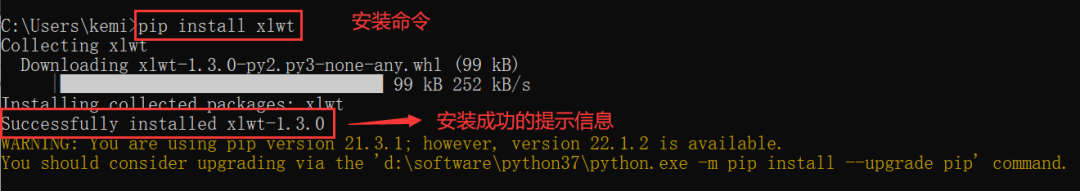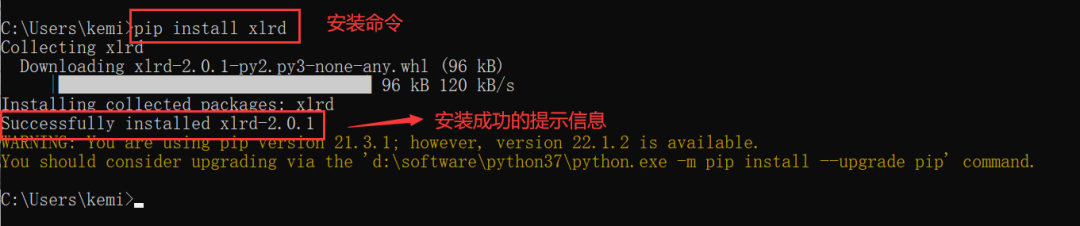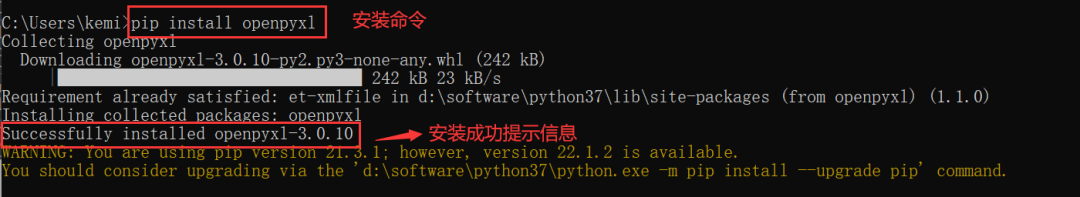## `二、xlwt库使用`

◈xlwt － 用于写入 Excel 文件，只支持.xls格式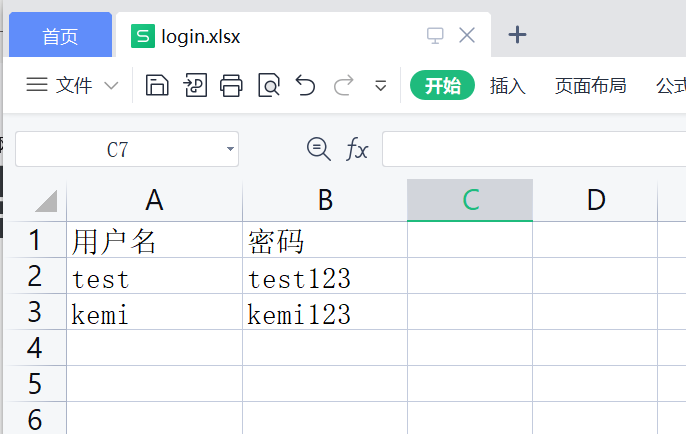2.使用xlwt写入数据的步骤

``````1）导包：import xlwt
2）创建一个文件对象:book=xlwt.Workbook()
4）添加内容:sh1.write(row,col,value)  #单元格行和列分别从0开始
5）保存文件:book.save(filename)
``````

3.代码实现

``````# coding = utf-8
import xlwt

#创建一个excel文件对象
book = xlwt.Workbook()
#添sheet工作表
sh1.write(0,0,'用户名') # 在A1单元格写入数据
sh1.write(0,1,'密码')   # 在B1单元格写入数据

row1 = ['test','test123']
# 结合循环写入一行数据
for i in range(len(row1)):
sh1.write(1,i,row1[i])

```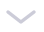```

## `三、xlrd库使用`

◈xlrd － 用于读取 Excel 文件，支持.xls和.xlsx格式

2.使用xlrd读取数据的步骤

``````1)导包：import xlrd
2)打开一个文件：book=xlrd.open_workbook(filename)
3)使用sheet工作表：sh1=book.sheet_by_name(sheet_name)
4)读取sheet工作表的属性信息
print('sheet总行数',sh1.nrows)
print('sheet总列数',sh1.ncols)
5)读取sheet工作表存储的文本内容
1）读取一行：row1=sh1.row_values(row) # 行号从0开始
2）读取一列：col1=sh1.col_values(col) # 列号从0开始
3）读取一个单元格：cell_value=sh1.cell(row,col).value
``````

3.代码实现

``````# coding = utf-8
import xlrd

sh1 = book.sheet_by_name('登录数据')
# 读取第一行的数据
row1 = sh1.row_values(0)
print('第一行数据：',row1)

# 读取第一列的数据
col1 = sh1.col_values(0)
print('第一列数据：',col1)

# 读取指定单元格的数据
cell = sh1.cell(1,1).value
print('A2单元格的值：',cell)

# 读取所有的数据
rows = sh1.nrows  # 获取当前工作表总的行数
for i in range(rows):
print('所有数据打印，第{}行,数据为：{}：'.format(i,sh1.row_values(i)))
``````

4.代码运行结果展示：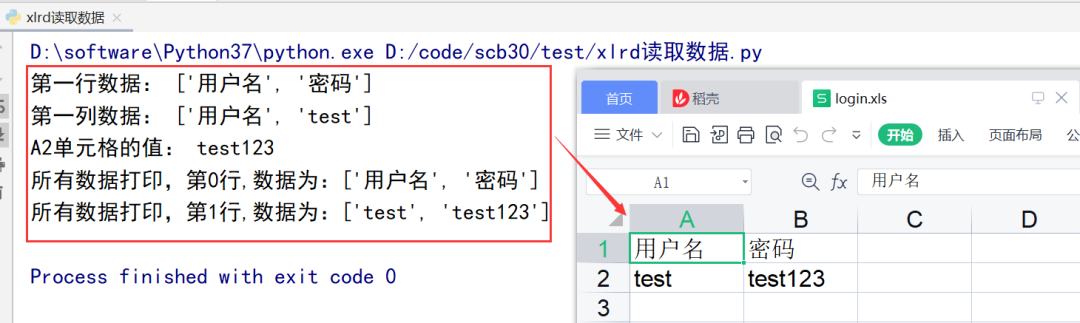## `四、openpyxl库使用--写入数据`

◈openpyxl － 既可以读文件、也可以写文件、也可以修改Excel文件；但是不支持 xls 格式

1.需求：对已存在的test_api.xlsx文件写入接口测试结果，如下图所示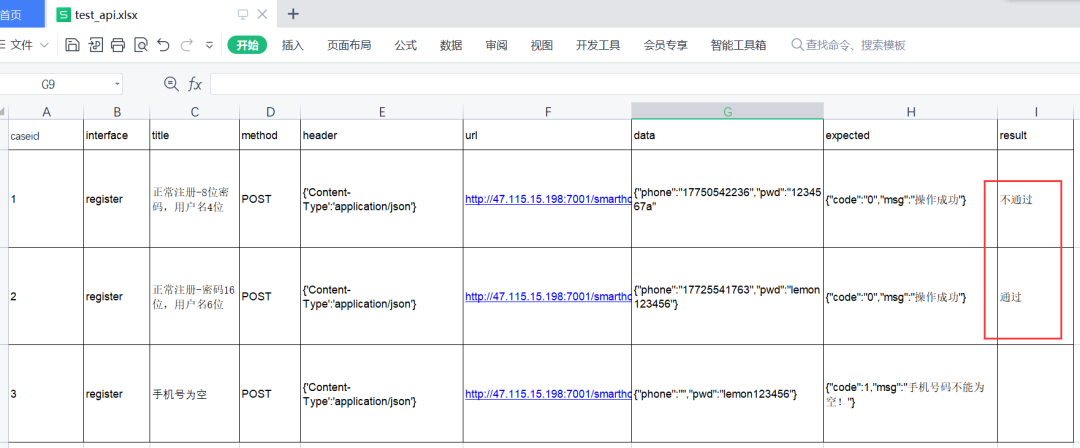2.使用openpyx写入数据的步骤

``````1）导包:import openpyxl
3）使用sheet工作表：sheet = book[sheetname]
4) 单元格写入：sh1['F2'] = 'PASS'  或者 sh1.cell(row,col).value='FAIL'  #行和列的索从1开始
6：保存文件:book.save(filename)
``````

3.代码实现

``````# coding = utf-8
import openpyxl

# 打开excel文件
# 通过工作表名字打开工作表
sh1 = book['register']
# 通过单元格的名称写入数据
sh1['I2'] = '不通过'
# 通过单元格的行、列写入数据
sh1.cell(3,9).value = '通过'
# 保存文件
book.save('test_api.xlsx')
``````

## `五、openpyxl库使用--读取数据`

2.使用openpyx读取数据的步骤

``````1）导包:import openpyxl
3）使用sheet工作表：sheet = book[sheetname]
4）读取sheet工作表的属性信息
返回工作表的最大行数：sheet.max_row
返回工作表的的最大列数:sheet.max_column
5)读取sheet工作表存储的文本内容
1）按单元格读取：cell1 = sh1['A1'].value 或者 cell2= sh1.cell(row,col).value #行和列的索引值是从1开始的
2) 按行读取
for row in sheet.iter_rows(max_row=3):# 读取前3行数据
for cell in row:
print(cell.value,end='\t')
print()
``````

3.代码实现

``````# coding = utf-8
import openpyxl

# 读取单元格数据
cell1 = sh1['A1'].value
print('A1单元格的值为：',cell1)
cell2 = sh1.cell(1,2).value
print('B1单元格的值为：',cell2)

# 读取前2行数据
print('读取前2行数据：')
for row in sh1.iter_rows(max_row= 2): # 读取前2行数据
for cell in row:
print(cell.value,end='\t|\t') # 不换行输出这一行中每个单元格的值
print() # 输出完一行之后换行

# 读取所有的数据
print('读取所有的数据：')
rows = sh1.max_row  # 获取当前工作表总的行数
for row in sh1.iter_rows(max_row=rows): # 读取所有的数据
for cell in row:
print(cell.value, end='\t|\t') # 不换行输出这一行中每个单元格的值
print() # 输出完一行之后换行
``````

4、运行结果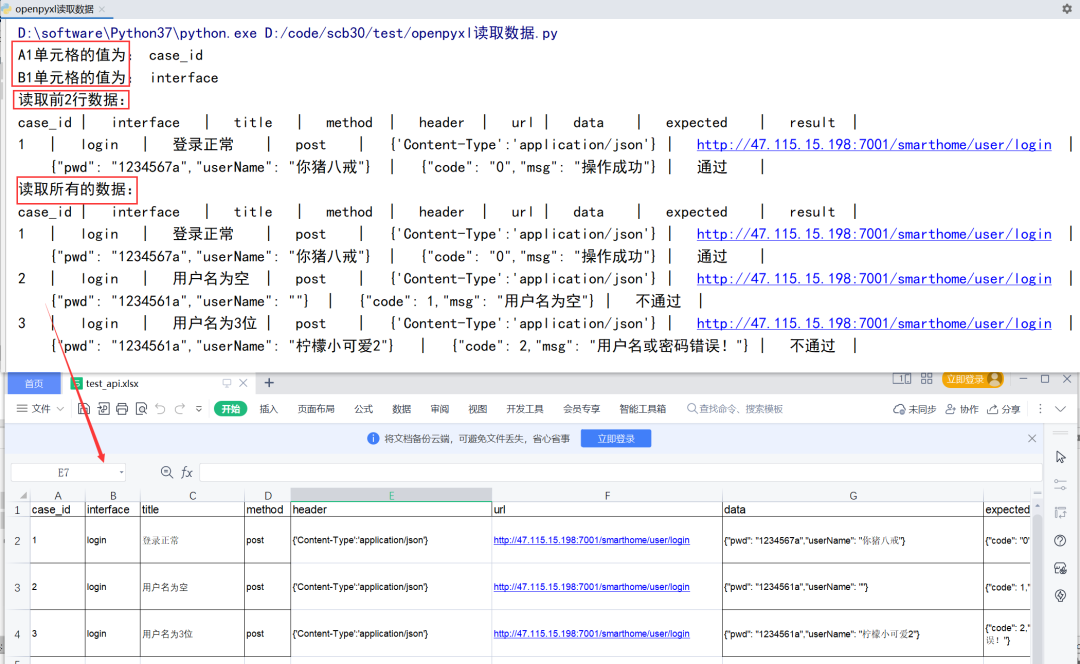## 软件测试工程师自学教程：

Jmeter实战讲解案例 — 软件测试人必会15 0

pdf(new) 更多>